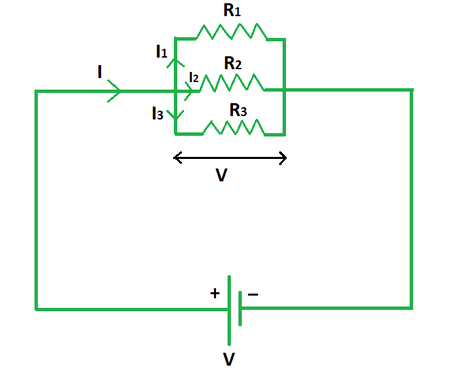# Resistors in Parallel Formula

• Last Updated : 15 May, 2022

Resistors in parallel are defined as a circuit in which two or more resistors are connected parallelly to each other such that both terminals of one resistor are linked to each terminal of the other resistor or resistors. A parallel circuit is also known as a current divider as the current of the circuit in a parallel resistor network can travel more than one channel since there are several pathways for it. As a result, the current is not the same along all of the parallel network’s branches. The voltage drop across all resistors in a parallel resistive network, on the other hand, is constant.Formula

The total resistance of a parallel circuit is evaluated by calculating the equivalent resistance of the circuit. Its formula states that the reciprocal of the equivalent resistance equals the sum of the reciprocals of individual resistances connected in parallel. The equivalent resistance is denoted by the symbol Req. Its SI unit of measurement is the ohm (Ω) and the dimensional formula is given by [M1L2A−2T−3].

1/Req = 1/R1 + 1/R2 + 1/R3 + ….. + 1/Rn

where,

Req is the equivalent resistance,

R1 is the resistance of the first resistor,

R2 is the resistance of the second resistor,

R3 is the resistance of the third resistor and so on.

Derivation

Suppose we have a circuit of voltage V and current I. The resistors R1, R2 and R3 are connected in parallel to the circuit.

It is known that in a parallel circuit, the current gets divided into number of parts which is equal to the number of resistors.

Let I1, I2 and I3 be the values into which the current I gets divided. We know,

I = I1 + I2 + I3

Dividing both sides by V we get,

I/V = I1/V + I2/V + I3/V

1/(V/I) = 1/(V/I1) + 1/(V/I2) + 1/(V/I3)

According to Ohm’s law, V = IR. So, the above equation becomes,

1/Req = 1/R1 + 1/R2 + 1/R3

This derives the formula for equivalent resistance in a parallel circuit.

### Sample Problems

Problem 1. Calculate the equivalent resistance if three resistances of 5 Ω, 2 Ω and 7 Ω are connected in parallel.

Solution:

We have,

R1 = 5 Ω

R2 = 2 Ω

R3 = 7 Ω

Using the formula we get,

1/Req = 1/R1 + 1/R2 + 1/R3

1/Req = 1/5 + 1/2 + 1/7

1/Req = 1/1.2

Req = 1.2 Ω

Problem 2. Calculate the equivalent resistance if three resistances of 2 Ω, 1 Ω and 3 Ω are connected in parallel.

Solution:

We have,

R1 = 2 Ω

R2 = 1 Ω

R3 = 3 Ω

Using the formula we get,

1/Req = 1/R1 + 1/R2 + 1/R3

1/Req = 1/2 + 1/1 + 1/3

1/Req = 1/0.55

Req = 0.55 Ω

Problem 3. Calculate the equivalent resistance if three resistances of 4 Ω, 6 Ω and 10 Ω are connected in parallel.

Solution:

We have,

R1 = 4 Ω

R2 = 6 Ω

R3 = 10 Ω

Using the formula we get,

1/Req = 1/R1 + 1/R2 + 1/R3

1/Req = 1/4 + 1/6 + 1/10

1/Req = 1/1.9

Req = 1.9 Ω

Problem 4. Calculate the equivalent resistance if three resistances of 5 Ω, 3 Ω and 8 Ω are connected in parallel.

Solution:

We have,

R1 = 5 Ω

R2 = 3 Ω

R3 = 8 Ω

Using the formula we get,

1/Req = 1/R1 + 1/R2 + 1/R3

1/Req = 1/5 + 1/3 + 1/8

1/Req = 1/1.5

Req = 1.5 Ω

Problem 5. Find the unknown resistance if three resistances of 3 Ω, 8 Ω and x Ω are connected in parallel to give an equivalent resistance of 1.8 Ω.

Solution:

We have,

R1 = 3 Ω

R2 = 8 Ω

Req = 1.8 Ω

Using the formula we get,

1/Req = 1/R1 + 1/R2 + 1/R3

=> 1/1.8 = 1/3 + 1/8 + 1/R3

=> 1/R3 = 1/1.8 – 1/3 – 1/8

=> 1/R3 = 1/11

=> R3 = 11 Ω

Problem 6. Find the unknown resistance if three resistances of 2.5 Ω, 1.5 Ω and x Ω are connected in parallel to give an equivalent resistance of 0.76 Ω.

Solution:

We have,

R1 = 2.5 Ω

R2 = 1.5 Ω

Req = 0.76 Ω

Using the formula we get,

1/Req = 1/R1 + 1/R2 + 1/R3

=> 1/0.76 = 1/2.5 + 1/1.5 + 1/R3

=> 1/R3 = 1/0.76 – 1/2.5 – 1/1.5

=> 1/R3 = 1/4

=> R3 = 4 Ω

Problem 7. Find the unknown resistance if three resistances of 3 Ω, 6 Ω and x Ω are connected in parallel to give an equivalent resistance of 1.6 Ω.

Solution:

We have,

R1 = 3 Ω

R2 = 6 Ω

Req = 1.6 Ω

Using the formula we get,

1/Req = 1/R1 + 1/R2 + 1/R3

=> 1/1.6 = 1/3 + 1/6 + 1/R3

=> 1/R3 = 1/1.6 – 1/3 – 1/6

=> 1/R3 = 1/8

=> R3 = 8 Ω

My Personal Notes arrow_drop_up